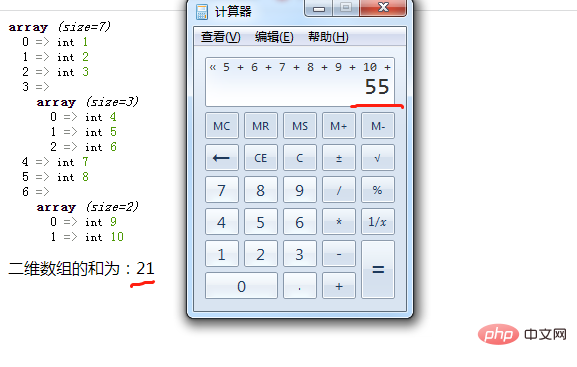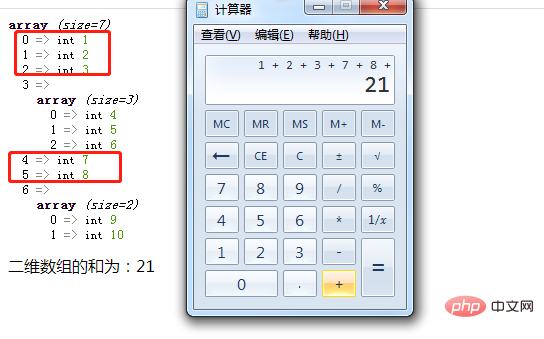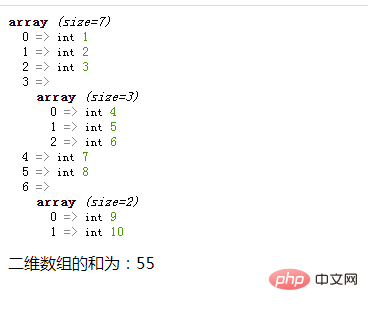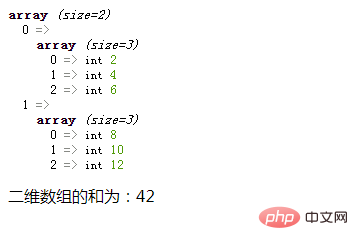# php二维数组求和用array_sum吗

php中二维数组求和只用array_sum()是不行的，因为array_sum()函数只处理一维数组；想要使用array_sum()实现二维数组求和，还需要借助foreach语句，语法“\$sum=0;foreach(\$arr as \$v){if(is_array(\$v)){\$sum+=array_sum(\$v);}else{\$sum+=\$v;}}”。php入门到就业线上直播课：进入学习
API 文档、设计、调试、自动化测试一体化协作工具：点击使用

php二维数组只用array_sum()是无法求和的。

`登录后复制````foreach(\$arr as \$v){ //循环体代码 }登录后复制```
• 遍历给定的 \$arr 数组，在每次循环中将当前外层数组的值赋给 \$v。

• 如果不是数组类型，则将不是的外层元素相加求和

• 如果是数组类型，则使用array_sum()函数计算该内层数组中所有元素的和，并与外层元素和相加在一起即可。

```if(is_array(\$v)){ \$sum+=array_sum(\$v); }else{ \$sum+=\$v; }登录后复制```

```function f(\$arr){ \$sum=0; foreach(\$arr as \$v){ if(is_array(\$v)){ \$sum+=array_sum(\$v); }else{ \$sum+=\$v; } } echo "二维数组的和为：".\$sum.""; }登录后复制```

```\$arr = array(1,2,3,array(4,5,6),7,8,array(9,10)); var_dump(\$arr); f(\$arr);登录后复制``````\$arr = array(array(2,4,6),array(8,10,12)); var_dump(\$arr); f(\$arr);登录后复制```• 相关标签：php php数组
• 程序员必备接口测试调试工具：点击使用

Apipost = Postman + Swagger + Mock + Jmeter

Api设计、调试、文档、自动化测试工具

后端、前端、测试，同时在线协作，内容实时同步

支持grpc,http,websocket,socketio,socketjs类型接口调试相关文章

相关视频

## 专题推荐

官方公众号

php中文网课程

扫码关注官方公众号
回复“phpcn01”领取php学习资料课程
全套工具、电子书、脑图、内部课程等